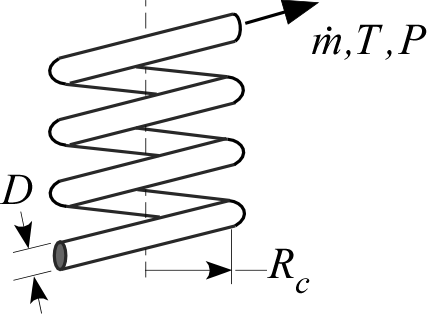Contents - Index

HelicalPipeFlowProcedure HelicalPipeFlow( Fluid\$, T, P, m_dot, D, L,RelRough,R_c: h_T, h_H, DELTAP, Nusselt_T, f, Re) returns lower and upper bounds on the average heat transfer coefficient and the pressure drop for a specified mass flow rate (m_dot) through a circular tube of diameter D and length L that is wound in a helix with radius R_c. The procedure modifies the results for a straight tube (obtained using the procedure PipeFLow) as discussed in the Heat Exchanger Design Handbook and Barron (1999). Properties are evaluated at the bulk temperature T and pressure P, for all built-in fluids except brines, for which the concentration in mixtures with water is provided in % in place of pressure. Units of the inputs and outputs depend upon the unit settings in EES.

Inputs:

Fluid\$ can be any fluid in the EES database. The fluid can be an ideal gas, a real fluid, a brine, or an incompressible fluid.

T - the bulk temperature of the fluid in [C], [K], [F], or [R].

P - pressure can be in [Pa], [kPa], [bar], [MPa], [atm], or [psia]. (Note that for brines, this parameter provides the concentration in %)

m_dot - mass flow rate in [kg/s], or [lbm/hr]

D - diameter of the tube in [m] or [ft]

L - length of the tube in [m] or [ft]

RelRough - the ratio of the dispersions on the wall of the tube to the tube diameter (must be between 0 and 0.05)

R_c - the radius of the helical winding in [m] or [ft]

Outputs (all but the first output are optional):

h_T - heat transfer coefficient in [W/m^2-K] or [Btu/hr-ft^2-R] assuming that the pipe wall is at constant temperature (lower bound)

h_H - heat transfer coefficient in [W/m^2-K] or [Btu/hr-ft^2-R] assuming a constant heat flux at the pipe wall (upper bound)

DELTAP - pressure difference between the inlet and outlet of the pipe in the pressure units set in the EES Unit System dialog

Nusselt_T - Nusselt number (determined for a constant temperature wall) [-]

f - friction factor [-]

Re - Reynolds number [-]

Notes

This procedure calculates the Reynolds and Prandtl numbers and then calls the procedure HelicalPipeFlow_N.   The procedure HelicalPipeFlow_N calls the straight tube procedure PipeFlow_N and modifies the results to account for the curvature.  PipeFlow_N will determine if the flow is laminar or turbulent. Transitional flow is assumed to occur for Reynold's numbers between 2300 and 3000 and interpolation is applied between the laminar and turbulent correlations. The ratio of L/D is used to apply a developing flow correction based on simultaneous hydrodynamic and thermal development; set L to a large number if developing flow corrections are not applicable.  The straight tube friction factor is modified as discussed in the Heat Exchanger Design Handbook (2008) in order to account for curvature.  The straight tube Nusselt number is modified as discussed in Barron (1999) in order to account for curvature.

Two values of the heat transfer coefficient are returned. The first, h_T is determined assuming that the wall is at constant temperature. The second is determined assuming that the wall is subjected to a constant heat flux. For laminar flow, these values should provide lower and upper bounds on the heat transfer coefficient. For turbulent flow, these values are identical.

Example 1:

\$UnitSystem SI Pa J K m

Fluid\$='Water'

T=300 [K]

P=200000 [Pa]

m_dot=1 [kg/s]

D=0.05 [m]

L=5 [m]

RelRough=0.001 [-]

R_c=0.2 [m]

CALL HelicalPipeFlow( Fluid\$, T, P, m_dot, D, L,RelRough,R_c: h_T, h_H, DELTAP,Nusselt_T,f,Re)

{Solution:

DELTAP=489.8[Pa]

f=0.03764 [-]

h_H=4176 [W/m^2-K]

h_T=4176 [W/m^2-K]

Nusselt_T=349.3 [-]

Re=29811 [-]

}

NOTE:  Starting with version 9.513, it is not necessary to supply all of the outputs to a Procedure.  If, for example, you only want to return output h_H, the call statement can appear as:

CALL HelicalPipeFlow( Fluid\$, T, P, m_dot, D, L,RelRough,R_c: , h_H, , , ,)

Example 2:

\$UnitSystem SI Pa K J

T=363 [K] "average temperature of fluid in duct"

C=50[%] "concentration of the propylene glycol-water solution"

m_dot=0.10 [kg/s] "flow rate"

D=0.025 [m] "inner pipe diameter"

L=5 [m] "length"

RelRough=0.005 [-] "relative roughness"

R_c=0.2 [m]

CALL HelicalPipeFlow('PG', T, C, m_dot, D, L,RelRough,R_c: h_T, h_H, DELTAP,Nusselt_T,f,Re)

{Solution:

DELTAP=210.7 [Pa]

f=0.05013

h_H=1173 [W/m^2-K]

Nusselt_T=73.95

Re=5623}